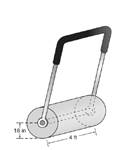Chapter 9.3, Problem 49E### Elementary Geometry for College St...

6th Edition
Daniel C. Alexander + 1 other
ISBN: 9781285195698

#### Solutions

Chapter
Section### Elementary Geometry for College St...

6th Edition
Daniel C. Alexander + 1 other
ISBN: 9781285195698
Textbook Problem
54 views

# A lawn roller in the shape of a right circular cylinder has a radius of length 18 in. and a length (height) of 4 ft. Find the area rolled during one complete revolution of the roller. Use the calculator value of π , and give the answer to the nearest square foot.To determine

To find:

The area rolled during one complete revolution of the roller.

Explanation

Given:

A lawn roller in the shape of a right circular cylinder has a radius of 18 in. and a length (height) of 4 ft.

Formula used:

The lateral area of the circular cylinder is L=2πrh

Calculation:

Let r be the radius and h be the altitude of the circular cylinder.

We have r=18 in

r=1812 ft=1.5 ft

We have r=1.5,h=4

Substitute the values in L=2πrh

### Still sussing out bartleby?

Check out a sample textbook solution.

See a sample solution

#### The Solution to Your Study Problems

Bartleby provides explanations to thousands of textbook problems written by our experts, many with advanced degrees!

Get Started

#### In Exercises 516, evaluate the given quantity. log4116

Finite Mathematics and Applied Calculus (MindTap Course List)

#### In Exercises 2340, find the indicated limit. 40. limx3xx2+72x2x+3

Applied Calculus for the Managerial, Life, and Social Sciences: A Brief Approach

#### Evaluate the integral. 35. /2/2x1+cos2xdx

Single Variable Calculus: Early Transcendentals

#### Using a binomial series, the Maclaurin series for is:

Study Guide for Stewart's Single Variable Calculus: Early Transcendentals, 8th

#### Sometimes, Always, or Never: fxy = fyx.

Study Guide for Stewart's Multivariable Calculus, 8th